# Electronics and Communication Engineering - Automatic Control Systems

Exercise : Automatic Control Systems - Section 1
16.
First column elements of Routh's tabulation are 3, 5,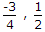, 2. It means that there
is one root in left half s plane
are two roots in left half s plane
are two roots in right half s plane
is one root in right half s plane
Explanation:

Since there are two sign changes in the first column, hence two roots in right half plane.

17.
When a unit step voltage drives a lag network the output
remains constant at unit step value
increases exponentially from zero to final value
decreases exponentially from 1 to 0
either (b) of (c) depending on values of R and C
Explanation:

At t = 0 the capacitor behaves as a short-circuit and output voltage is 0. At t = ∞ capacitor behaves as an open-circuit and V0 = Vi.

18.
For the given figure C(s)/R(s)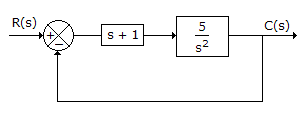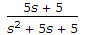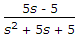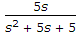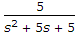Explanation: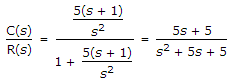.

19.
For the circuit in the given figure,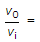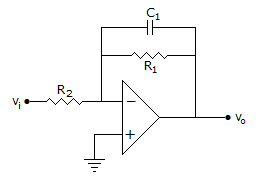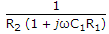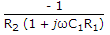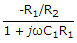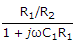Explanation: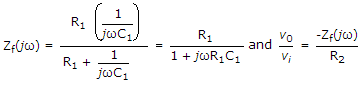.

20.
A system has its two poles on the negative real axis and one pair of poles lies on jω axis. The system is
stable
unstable
limitedly stable
either (a) or (c)